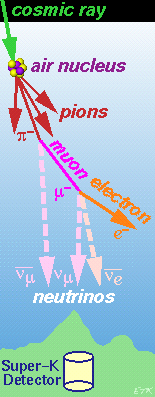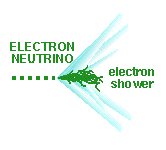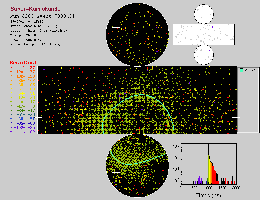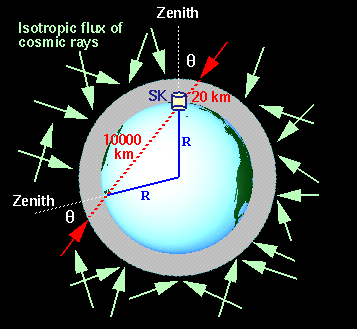#ATMOSPHERIC NEUTRINOS

ATMOSPHERIC NEUTRINOS are produced in the collision of primary cosmic rays (typically protons) with nuclei in the upper atmosphere. This creates a shower of hadrons, mostly pions. The pions decay to a muon and a muon neutrino. The muons decay to an electron, another muon neutrino, and an electron neutrino. Based on this simple kinematic chain, one predicts a flux ratio of 2-to-1 muon neutrinos to electron neutrinos. This is modified somewhat in detailed calculations: there are also kaons produced in the hadronic shower, and at high energy, some muons live long enough (due to Lorentz time dilation) that they are stopped in the earth before decaying in flight to high energy neutrinos. However, calculating the flux ratio in this way does cancel out many uncertainties, principally the absolute flux of incoming cosmic rays, and the yield of hadrons in high energy collisions. The final estimated uncertainty in the flux ratio is about 5%.

Super-Kamiokande detects atmospheric neutrinos by their interaction with the nuclei of hydrogen and oxygen in the 22.5 kiloton central fiducial mass of water. The products of this reaction are usually "fully contained" in the detector volume. The neutrino flavor is tagged by detecting and identifying the final state lepton (muon or electron). Low energy (E < 1 GeV) neutrinos typically react by quasi-elastic scattering, with only the lepton visible in the final state. At higher energies, the final state muon or electron is often accompanied by a pion (single pi production) or numerous hadrons (deep inelastic scattering). To properly associate the event with the neutrino type, we therefore select events with a single Cherenkov ring. The one exception are so-called "partially contained" events, where a particle exits the central volume into the outer detector. These are identified as muon neutrinos (with 98% accuracy), because only muons have such great penetrating power.

Fully contained muons and electrons are identified by a pattern recognition algorithm that uses the maximum likelihood method to compare the measured distribution of hits and with the expected shape due to a muon (sharp, collapsed angle if beta < 1) and an electron (fuzzy due to the electromagnetic shower).click event display to see full size ---->click event display to see full-size ---->In addition to tagging the event as coming from a muon or electron neutrino, we can estimate properties from the Cherenkov ring. We fit the direction of the ring, which corresponds to the direction of the outgoing electron or muon. But this direction is correlated with the direction of the neutrino. At high energy, the lepton direction is correlated to within 15 degrees with the neutrino direction. In addition, the amount of Cherenkov light is a measure of the energy of the lepton. Again, this is correlated with the energy of the neutrino.

By measuring the number of events of each type, as a function of energy and direction, we can find out if neutrino oscillations are affecting the results. Briefly speaking: neutrino oscillations can effect the number of neutrinos detected as a function of distance travelled. The distance travelled is determined
by the arrival angle with respect to the vertical (zenith).

The first piece of evidence for neutrino oscillations is that we measure an anomalous number of muon neutrino events compared to electron neutrino events. As described above, the ratio of muon to electron neutrinos is reasonably well predicted, based on the simple kinematics of pion decay. In our publication: (Phys.Rev.Lett.81(1998)p.1562, hep-ex/9807003) we reported the double-ratio:

 (N_mu / N_e )_data ------------------ =   0.63 +- .03 (statistical) +- .05 (systematic) (N_mu / N_e)_predicted

If the data agreed with the standard prediction of muon and electron neutrino production, this number should be about 1. However, the measured number is much less than one.The second piece of evidence for neutrino oscillations is that we measure a significant up-down asymmetry of high energy muon neutrino events. Above a few GeV, atmospheric neutrinos can be traced back to primary cosmic rays of energy greater than 10 GeV. Above this energy, the primary cosmic rays are not deflected very much by the earth's magnetic field. Cosmic rays arrive at the earth almost isotropically, having been randomized by interstellar magnetic fields. This results in the prediction that the flux of atmospheric neutrinos should be equal for equal angles with the zenith and nadir. Another way of saying this is that the cosine(zenith) distribution should be symmetric. We quantify this be calculating the up/down asymmetry: (A = UP-DOWN/UP+DOWN). For high energy muon neutrino events, we measured an asymmetry of:

A = 0.296 +- 0.048(statistical) +- 0.01(systematic).

The asymmetry was consistent with zero for the predicted value, so the above result is greater than 6 standard deviations from expectation. Furthermore, the up/down asymmetry was consistent with zero for high energy electron neutrinos. This can be dramatically seen in the zenith angle distribution of high energy neutrino events. Here, cosine(zenith) near -1 is upgoing, and the neutrinos have travelled a long distance of the order of 10,000 kilometers.  Cosine(zenith) near +1 is downgoing, and the neutrinos have travelled a short distance of the order of 10-100 kilometers. This graph is a preliminary result based on the current full data-set; details of the analysis of high energy events based on the first 414 days of data
were published in Phys. Lett. B436(1998)p.33 (hep-ex/9805006).The third piece of evidence, used to confirm the above result, came from an independent data set. Muon neutrinos also interact in the rock around and below the detector, with the final state muon entering the detector. If the neutrino and muon come from above, these events are lost in the constant background rain of cosmic ray muons. But cosmic rays can't penetrate the earth from below, so any entering muon must be neutrino induced. We studied these so-called upward-going muons and found that the rate of low and high energy events, and their angular distribution, were in poor agreement with the standard prediction, but in good agreement with a hypothesis of neutrino oscillations. Our first paper on upward-going muons was published in Phys.Rev.Lett.82(1999)p.2644 (hep-ex/9812014).

As you can see, much of our evidence comes from a strong deviation between data and expectation as a function of zenith angle. We have built our detector to be as uniform as possible, and we estimate that it is up-down symmetric in response to better than a few percent. For comparison, we also study the azimuthal dependence of the data. Because the distance of neutrino travel is constant as a function of azimuth, no distortion is expected due to neutrino oscillations. At high energies, the data are flat as a function of azimuth, as expected. At lower energies, however, we have observed an asymmetry that can be traced to an effect where the earth's magnetic field deflects incoming cosmic rays. This is a well-known phenomenon, called the East-West Effect, originally discovered in the 1930's and used to infer that incoming cosmic rays are positively charged. Accounting for this effect, again, our data agrees well with expectation. In terms of neutrino oscillations, this null result is interesting because it implies that the detailed flux calculations we rely on for the predicted zenith angle distribution properly account for the geomagnetic field.

Further information:

Boston University Thesis by Mark Messier: Evidence for Neutrino Mass from Observations of Atmospheric Neutrinos with Super-Kamiokande

General interest article, Neutrino Oscillations, published in American Scientist magazine by Kate Scholberg and Kenji Kaneyuki.

Next month (Aug 1999): Detecting Massive Neutrinos in Scientific American, by Edward Kearns, Takaaki Kajita and Yoji Totsuka.

A hypertext page at U.C.I. about neutrinos, by Dave Casper, and another at U.Hawaii by John Learned.

The Neutrino Oscillation Industry, a set of links to other experiments and topics maintained by Maury Goodman of A.N.L.

Back to Super-K at Boston U.

ETK